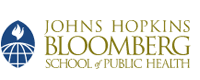## Johns Hopkins University, Dept. of Biostatistics Working Papers

Published in Biometrics 62(1):61-65

#### Abstract

The objective of this paper is to develop a hypothesis testing procedure to determine whether a common source outbreak has ended. We do not assume that the calendar date of exposure to the pathogen is known. We assume an underlying parametric model for the incubation period distribution of a 2-paramter exponential model with a guarantee time, although the parameters are not assumed to be known. The hypothesis testing procedure is based on the spacings between ordered calendar dates of disease onset of the cases. A simulation study was performed to evaluate the robustness of the methods to a lognormal model for the incubation period of infectious diseases. We investigated whether multiple testing over the course of the outbreak could increase the overall outbreak-wise type 1 error probability. We derive expressions for the outbreak-wise type 1 error probability and show that multiple testing has minimal effect on inflating that error probability.

#### Disciplines

Disease Modeling | Statistical Methodology | Statistical Theory

COinS